# US5189557A - High variable magnification range zoom lens - Google Patents

## Info

Publication number
US5189557A
US5189557A US07/793,094 US79309491A US5189557A US 5189557 A US5189557 A US 5189557A US 79309491 A US79309491 A US 79309491A US 5189557 A US5189557 A US 5189557A
Authority
US
United States
Prior art keywords
sub
lens unit
lt
zoom lens
refractive power
Prior art date
Legal status (The legal status is an assumption and is not a legal conclusion. Google has not performed a legal analysis and makes no representation as to the accuracy of the status listed.)
Application number
US07/793,094
Inventor
Hiroshi Endo
Current Assignee (The listed assignees may be inaccurate. Google has not performed a legal analysis and makes no representation or warranty as to the accuracy of the list.)
Canon Inc
Original Assignee
Canon Inc
Priority date (The priority date is an assumption and is not a legal conclusion. Google has not performed a legal analysis and makes no representation as to the accuracy of the date listed.)
Filing date
Publication date
Priority to JP2-316261 priority Critical
Priority to JP2316249A priority patent/JP2744348B2/en
Priority to JP2-316249 priority
Priority to JP2-316248 priority
Priority to JP31624890A priority patent/JPH04186211A/en
Priority to JP31626190A priority patent/JPH04186213A/en
Application filed by Canon Inc filed Critical Canon Inc
Assigned to CANON KABUSHIKI KAISHA A CORP. OF JAPAN reassignment CANON KABUSHIKI KAISHA A CORP. OF JAPAN ASSIGNMENT OF ASSIGNORS INTEREST. Assignors: ENDO, HIROSHI
Application granted granted Critical
Publication of US5189557A publication Critical patent/US5189557A/en
Anticipated expiration legal-status Critical
Application status is Expired - Lifetime legal-status Critical

• 239000003570 air Substances 0 abstract claims description 11
• 238000000926 separation method Methods 0 abstract description 23
• 230000003287 optical Effects 0 claims description 6
• 230000004075 alteration Effects 0 description 25
• 230000000694 effects Effects 0 description 5
• 230000035945 sensitivity Effects 0 description 4
• 238000004904 shortening Methods 0 description 4
• 239000002131 composite material Substances 0 description 3
• 230000001965 increased Effects 0 description 3
• 238000006243 chemical reaction Methods 0 description 1
• 230000002349 favourable Effects 0 description 1
• 239000011521 glass Substances 0 description 1
• 230000036629 mind Effects 0 description 1
• 239000010950 nickel Substances 0 description 1
• 238000005365 production Methods 0 description 1
• 230000001603 reducing Effects 0 description 1
• 230000011514 reflex Effects 0 description 1
• 238000007514 turning Methods 0 description 1

## Images

•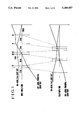•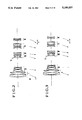•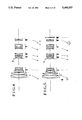•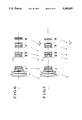••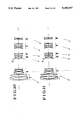•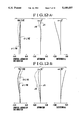•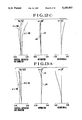•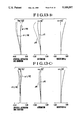•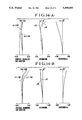••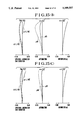•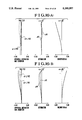•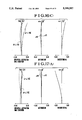•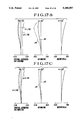•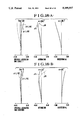•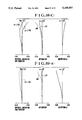•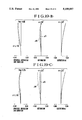•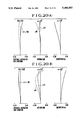•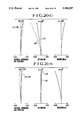•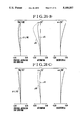## Classifications

• GPHYSICS
• G02OPTICS
• G02BOPTICAL ELEMENTS, SYSTEMS, OR APPARATUS
• G02B15/00Optical objectives with means for varying the magnification
• G02B15/14Optical objectives with means for varying the magnification by axial movement of one or more lenses or groups of lenses relative to the image plane for continuously varying the equivalent focal length of the objective
• G02B15/16Optical objectives with means for varying the magnification by axial movement of one or more lenses or groups of lenses relative to the image plane for continuously varying the equivalent focal length of the objective with interdependent non-linearly related movements between one lens or lens group, and another lens or lens group
• G02B15/163Optical objectives with means for varying the magnification by axial movement of one or more lenses or groups of lenses relative to the image plane for continuously varying the equivalent focal length of the objective with interdependent non-linearly related movements between one lens or lens group, and another lens or lens group having a first movable lens or lens group and a second movable lens or lens group, both in front of a fixed lens or lens group
• G02B15/167Optical objectives with means for varying the magnification by axial movement of one or more lenses or groups of lenses relative to the image plane for continuously varying the equivalent focal length of the objective with interdependent non-linearly related movements between one lens or lens group, and another lens or lens group having a first movable lens or lens group and a second movable lens or lens group, both in front of a fixed lens or lens group having an additional fixed front lens or group of lenses
• G02B15/173Optical objectives with means for varying the magnification by axial movement of one or more lenses or groups of lenses relative to the image plane for continuously varying the equivalent focal length of the objective with interdependent non-linearly related movements between one lens or lens group, and another lens or lens group having a first movable lens or lens group and a second movable lens or lens group, both in front of a fixed lens or lens group having an additional fixed front lens or group of lenses arranged +-+

## Abstract

A high variable magnification range zoom lens is disclosed, comprising, in the order from the object side, a first lens unit having a positive refractive power, a second lens unit having a negative refractive power, a third lens unit having a positive refractive power, a fourth lens unit having a negative refractive power, a fifth lens unit having a positive refractive power, and a sixth lens unit having a negative refractive power, wherein letting the air separation between the i-th lens unit and the (i+1)st lens unit in the wide-angle end and the telephoto end be denoted by DiW and DiT, respectively, zooming from the wide-angle end to the telephoto end is performed so as to satisfy the following conditions:
`D.sub.1W <D.sub.1T`
`D.sub.2W >D.sub.2T`
`D.sub.3W <D.sub.3T`
`D.sub.4W >D.sub.4T`

## Description

BACKGROUND OF THE INVENTION

1. Field of the Invention

This invention relates to zoom lenses suited to a single-lens reflex camera, video camera, etc. and, more particularly, to a zoom lens, while including the wide-angle region, having an extremely high variable magnification range of as much as 10.

2. Description of the Related Art

The zoom lenses including focal lengths of from the wide-angle to the telephoto region are known in, for example, Japanese Laid-Open Patent Applications No. Sho 57-164710 and No. Sho 60-39613. In these publications, zoom lenses composed of five units of plus, minus, plus, minus, plus, when counted from the object side, are obtained with a zoom ratio of 3 to 5.

Meanwhile, in Japanese Laid-Open Patent Application No. Hei 1-191819, likewise with the 5-unit form of plus, minus, plus, minus, plus, a zoom lens having a zoom ratio of about 10 is disclosed.

However, the above-described zoom lenses have their diameters of the front lens members (the first lens unit) and their total lengths of the optics (the distance from the first lens surface to the image plane) to get relatively large. Further, in particular, Japanese Laid-Open Patent Applications No. Sho 57-164710 and No. Sho 60-39613 are low in the zoom ratio, and could not be said to be sufficient, as, in recent years, a zoom lens having a higher zoom ratio is demanded. In Japanese Laid-Open Patent Application No. Hei 1-191819, a zoom lens of a remarkably high zoom ratio is disclosed, but the total length of the optics is long and the diameter of the front lens members, too, is considerably large.

SUMMARY OF THE INVENTION

With such problems in mind, an object of the present invention is to provide a zoom lens having a zoom ratio of about 10, while nevertheless having a total length of the optics shortened and a good optical performance.

To achieve the above-described object, a high variable magnification range zoom lens according to the invention comprises, in the order from the object side, a first lens unit having a positive refractive power, a second lens unit having a negative refractive power, a third lens unit having a positive refractive power, a fourth lens unit having a negative refractive power, a fifth lens unit having a positive refractive power and a sixth lens unit having a negative refractive power, wherein letting the air separation between the i-th lens unit and the (i+1)st lens unit in a wide-angle end and a telephoto end be denoted by DiW and DiT, respectively, zooming from the wide-angle end to the telephoto end is performed under the following conditions:

`D.sub.1W <D.sub.1T`

`D.sub.2W >D.sub.2T`

`D.sub.3W <D.sub.3T`

`D.sub.4W >D.sub.4T`
BRIEF DESCRIPTION OF THE DRAWINGS

FIG. 1 is a diagram showing the paraxial arrangement concerning the present invention.

FIG. 2 is a lens block diagram of a numerical example 1 concerning the invention.

FIG. 3 is a lens block diagram of a numerical example 2 concerning the invention.

FIG. 4 is a lens block diagram of a numerical example 3 concerning the invention.

FIG. 5 is a lens block diagram of a numerical example 4 concerning the invention.

FIG. 6 is a lens block diagram of a numerical example 5 concerning the invention.

FIG. 7 is a lens block diagram of a numerical example 6 concerning the invention.

FIG. 8 is a lens block diagram of a numerical example 7 concerning the invention.

FIG. 9 is a lens block diagram of a numerical example 8 concerning the invention.

FIG. 10 is a lens block diagram of a numerical example 9 concerning the invention.

FIG. 11 is a lens block diagram of a numerical example 10 concerning the invention.

FIGS. 12(A), 12(B) and 12(C) are graphs of the various aberrations of the numerical example 1 concerning the invention.

FIGS. 13(A), 13(B) and 13(C) are graphs of the various aberrations of the numerical example 2 concerning the invention.

FIGS. 14(A), 14(B) and 14(C) are graphs of the various aberrations of the numerical example 3 concerning the invention.

FIGS. 15(A), 15(B) and 15(C) are graphs of the various aberrations of the numerical example 4 concerning the invention.

FIGS. 16(A), 16(B) and 16(C) are graphs of the various aberrations of the numerical example 5 concerning the invention.

FIGS. 17(A), 17(B) and 17(C) are graphs of the various aberrations of the numerical example 6 concerning the invention.

FIGS. 18(A), 18(B) and 18(C) are graphs of the various aberrations of the numerical example 7 concerning the invention.

FIGS. 19(A), 19(B) and 19(C) are graphs of the various aberrations of the numerical example 8 concerning the invention.

FIGS. 20(A), 20(B) and 20(C) are graphs of the various aberrations of the numerical example 9 of concerning the invention.

FIGS. 21(A), 21(B) and 21(C) are graphs of the various aberrations of the numerical example 10 concerning the invention.

Incidentally, in the graphs of the aberrations, the graphs of the figure numbers with the suffixes (A), (B) and (C) are the graphs of the various aberrations of the wide-angle end, an intermediate focal length and the telephoto end, respectively, S stands for the sagittal image surface, M for the meridional image surface, d for the d-line and g for the g line.

DETAILED DESCRIPTION OF THE PREFERRED EMBODIMENTS

FIG. 1 to FIG. 11 show the lens block diagrams of the zoom lenses concerning the present invention. Particularly, FIG. 1 shows the paraxial arrangement and the optical paths of an on-axial light ray and an off-axial light ray in the zoom lens concerning the invention.

I denotes the first lens unit having a positive refractive power, II denotes the second lens unit having a negative refractive power, III denotes the third lens unit having a positive refractive power, IV denotes the fourth lens unit having a negative refractive power, V denotes the fifth lens unit having a positive refractive power and IV denotes the sixth lens unit having a negative refractive power. Zooming from the wide-angle end to the telephoto end is performed by tracing, on an optical axis, the motion loci shown by the dashed lines or arrows. That is, when the air separation between the i-th lens unit and the (i+1)st lens unit in the wide-angle end and the telephoto end are denoted by DiW and DiT, respectively, zooming from the wide-angle end to the telephoto end is performed by moving each lens unit, or by making part of the lens units stationary, so as to satisfy the following conditions:

`D.sub.1W <D.sub.1T`

`D.sub.2 W>D.sub.2 T`

`D.sub.3 W<D.sub.3 T`

`D.sub.4 W>D.sub.4 T`

Also, D5 W>D5 T is satisfied and further the sixth lens unit is made to move so as to position itself on the object side when in the position of the telephoto end as compared with the wide-angle end. Also, focusing is performed by moving the sixth lens unit. Also, S indicates a stop. The stop S is stationary relative to the third lens unit III.

In the present embodiment, at first, in the wide-angle end, the positive first lens unit and the negative second lens unit are made to come nearest to each other so that the composite refractive power (f12) of the first lens unit and the second lens unit becomes negative (f12 <0). Also, the separation between the second and third lens units is made to be largest, the separation between the third and fourth lens units is made to be smallest, and, on its image side, the fifth lens unit by a space and the sixth lens unit by a further space are arranged. With the first lens unit and the second lens unit, their composite refractive power becomes negative, and, with the positive third lens unit and the negative fourth lens unit, the refractive power becomes relatively weak, and further with the use of the positive fifth lens unit and the negative sixth lens unit, the entirety is formed into the retrofocus type. By arranging the negative sixth lens unit in such a way, the aim of shortening the back focal distance is fulfilled with the result of shortening the total length. Along with this, by the sixth lens unit VI, the off-axial light ray is bent upward with the result that the outer diameters of the fourth, fifth and sixth lens units are minimized.

Next, in the telephoto end, the separation between the positive first lens unit and the negative second lens unit is largest, the separation between the negative second lens unit and the positive third lens unit is smallest, and further the negative fourth lens unit, the positive fifth lens unit and the negative sixth lens unit are arranged in the most approached states to each other, so that, as a whole, the lens form is of high telephoto ratio. Thus, a shortening of the total length of the lens is attained.

Next, explanation is given to the conversion of the outer diameter of the lens to compact form. In general, in the high variable magnification range zoom lens including the wide-angle region, a lens group of the largest outer diameter is the first lens unit. Here, as shown in FIG. 1, as the elements of determining the outer diameter of the first lens unit, there are the off-axial light ray in the wide-angle end and the on-axial FNo light ray in the telephoto end. When the full-aperture F-number is denoted by FNo, the diameter of the on-axial FNO light ray by D, and the focal length of the entire lens system by f, the following formula is established:

`f.sub.No =f/D`

The effective diameter of the first lens unit in the telephoto end is automatically determined by the height of pass of the on-axial FNo light ray. On the other hand, the effective diameter in the wide-angle end including the wide-angle region depends on the off-axial light ray incident on the first lens unit. Because the inclination of this light ray is very large, it becomes difficult to lessen the outer diameter of the first lens unit to a small value while securing the off-axial light beam in the wide-angle end. To cope with this difficulty, in the present embodiment, the composite negative refractive power of the first lens unit and the second lens unit is made strong. In other words, the negative refractive power of the second lens unit is made strong. Also, the lens separation D2W in the wide-angle end is made as small as possible so that the off-axial light rays passes through as near a position of the first lens unit as possible.

By the way, when the negative refractive power of the second lens unit is made strong, the lens system becomes the retrofocus type, particularly, in the wide-angle end, so that the back focal distance becomes longer than necessary. The total length of the optics (the distance from the first lens surface to the image plane) tends to become long.

In the present invention, it is desirable that this tendency is canceled by letting the second lens unit and the sixth lens unit have such negative refractive powers as to satisfy the following conditions:

`0.1<|f.sub.6 /|f.sub.T 0.5               (1)`

and/or

`1.5<|f.sub.6 /f.sub.2 |<6.0              (2)`

where f2 and f6 are the focal lengths of the second lens unit and the sixth lens unit, respectively, and fT is the focal length of the entire lens system in the telephoto end. This leads to the aim of turning the total length to compact form. Also, to make small the lens separation D2W means to make small the amount of movement with zooming of the air separation of the second lens unit and the third lens unit. Therefore, the magnification varying effect of the third lens unit becomes small. But, in the present invention, the entire lens system is constructed with the six lens units and by making each of the lens units that follow the second lens unit to share the magnification varying effect, the aim of increasing the variable magnification range is attained.

The inequalities of condition (1) are to regulate the focal length of the sixth lens unit in relation to the longest focal length of the entire lens system. When the refractive power of the sixth lens unit gets stronger than the lower limit, the aberrations, particularly, distortion in the telephoto end, worsen. When the upper limit is exceeded, the magnification varying effect of the sixth lens unit becomes small. To satisfy the magnification variation ratio of the entire lens system, the amount of movement of each lens unit increases. It is not preferable to have the total length elongated. Further, more desirably, if a condition of D5W >D5T is satisfied, it is really good.

The inequalities of condition (2) are to regulate the focal length of the sixth lens unit in relation to the focal length of the second lens unit. When the refractive power of the sixth lens unit gets stronger than the lower limit, the tendency to the telephoto type over the entire lens system becomes strong. Therefore, although the total length becomes short, it becomes difficult to secure the necessary back focal distance. When the upper limit is exceeded, the tendency to the retrofocus type over the entire lens system becomes strong, causing the total length to increase objectionably.

Also, in the present invention, it is preferable to further satisfy the following conditions:

`0.26<f.sub.1 /f.sub.T <0.88                                (3)`

`0.11<|f.sub.2 |/f.sub.1 <0.31            (4)`

`0.08<f.sub.5 /f.sub.T <0.3                                 (5)`

where f1 and f5 are the focal lengths of the first lens unit and the fifth lens unit, respectively.

Further, it is preferable to satisfy the following conditions:

`1.1<f.sub.3 /|f.sub.2 |<3.7              (6)`

`0.15<(D.sub.1T -D.sub.1W)/f.sub.T <0.3                     (7)`

`0.07<(D.sub.2W -D.sub.2T)/f.sub.T <0.2                     (8)`

where f3 is the focal length of the third unit.

It is also preferable that the second lens unit has at least two negative lenses and at least one positive lens, the lens on the most object side being the negative lens, and, when the radii of curvature on the object side and the image side of this negative lens are denoted by r21 and r22, satisfies the following condition:

`|r.sub.22 /r.sub.21 |<0.23               (9)`

It is also preferable to form the fifth lens unit in such a manner that it has at least two positive lenses and at least one negative lens, the lens surface nearest to the object side being concave toward the object side.

Next, the significances of the above-described conditions are explained.

The inequalities of condition (3) are to regulate the focal length of the first lens unit in relation to the focal length of the entire lens system in the telephoto end. When the positive refractive power of the first lens unit gets stronger than the lower limit, it is advantageous for shortening the total length, but the outer diameter of the first lens unit becomes large to secure the off-axial light bundle in the wide-angle end, and further the aberrations become difficult to correct. When the positive refractive power of the first lens unit gets weaker than the upper limit, the outer diameter of the first lens unit becomes small, but the total length becomes objectionably long.

The inequalities of condition (4) are, under the condition (3), to regulate the focal length of the second lens unit in relation to the focal length of the first lens unit. When the negative refractive power of the second lens unit gets stronger than the lower limit, the outer diameter of the first lens unit can be made small, but the back focal distance becomes longer than necessary, causing the total length to become long. Further, the various aberrations which are produced in the second lens unit, particularly, spherical aberration and distortion, become difficult to correct in good balance. When the negative refractive power of the second lens unit gets weaker than the upper limit, it is favorable on the aberration correction, but the outer diameter of the first lens unit increases so that the compact form becomes difficult to achieve.

The inequalities of condition (5) are to regulate the focal length of the fifth lens unit in relation to the focal length of the entire lens system in the telephoto end. When the refractive power of the fifth lens unit gets stronger than the lower limit, it is advantageous for making the outer diameters of the fifth and sixth lens units to compact form, the various aberrations which are produced in the fifth lens unit become too large and those are difficult to correct by the other lens units. When the positive refractive power of the fifth lens unit gets weaker than the upper limit, the outer diameters of the fifth and sixth lens units increase, and the total length, too, becomes objectionably long.

The inequalities of condition (6) are to regulate the focal length of the third lens unit in relation to the focal length of the second lens unit. When the positive refractive power of the third lens unit gets stronger than the lower limit, it is advantageous for making the total length of the entire lens system and the outer diameters of the fourth, fifth and sixth lens units to compact form, but the various aberrations which are produced in the third lens unit become large and those are difficult to correct. When the positive refractive power of the third lens unit gets weaker than the upper limit, the total length of the entire lens system and the outer diameters of the fourth, fifth and sixth lens units become objectionably long.

The inequalities of condition (7) are to regulate the difference between the air separations in the telephoto end and the wide-angle end between the first and second lens units in relation to the focal length of the telephoto end, in other words, the amount of variation with zooming of the separation between the first and second lens units. When the amount of variation of the separation gets smaller than the lower limit, the magnification varying effect of the second lens unit becomes small. To obtain the prescribed ratio of magnification change, a necessity of varying the air separation between the other lens units arises. To take the space for this purpose in the wide-angle end, the total length of the entire lens system in the wide-angle end is caused to increase. When the amount of variation of the separation gets larger than the upper limit, the magnification varying effect of the second lens unit becomes large. It is advantageous for increasing the range of magnifications. However, to secure the sufficient off-axial light bundle in the telephoto end, the outer diameter of the first lens unit or the fourth, fifth and sixth lens units is caused to increase.

The inequalities of condition (8) are to regulate the difference between the air separations in the wide-angle end and the telephoto end between the second and third lens units, in other words, the amount of variation with zooming of the separation between the second and third lens units. When the amount of variation of the separation gets smaller than the lower limit, it is advantageous for reducing the outer diameter of the first lens unit to a minimum, but a necessity of largely varying the air separation between the fourth and fifth lens units arises. This space has to be previously secured in the wide angle end. The separation between the fourth lens unit and the fifth lens unit widens in the wide-angle end. To secure the off-axial light bundle, the outer diameter of the fifth lens unit is caused to increase. When the amount of variation of the separation gets larger than the upper limit, it is advantageous for increasing the range of magnifications, but means that the separation between the second and third lens units in the wide-angle end becomes large. To secure the off-axial light bundle, the outer diameter of the first lens unit becomes objectionably large.

Further, the second lens unit is a lens unit having a strong negative refractive power compared with the focal length of the entire lens system in the telephoto end. For this reason, to minimize the various aberrations which are produced in the second lens unit, it is preferable that the second lens unit is constructed with at least two negative lenses and at least one positive lens, and that, particularly, the negative lens nearest to the object side is made to satisfy the condition (9). The inequality of condition (9) is to regulate the ratio of the radii of curvature of the lens nearest to the object side of the second lens unit. That the radius r22 gets gentler than the upper limit as compared with the radius r21, means that the negative refractive power of the aforesaid negative lens becomes weak. For this reason, to let the second lens unit have a prescribed refractive power, the radius of curvature of the other negative lens would become tight, or the number of lens elements would increase. In the former case, production of the various aberrations becomes remarkable. In the latter case, the second lens unit becomes thick. To secure the off-axial light bundle in the wide-angle end, therefore, the outer diameter of the first lens unit is caused to increase.

By the way, in the present invention, the diameter of the first lens unit is minimized and, at the same time, focusing is performed by moving the sixth lens unit having the negative refractive power along the optical axis in order that the focusing can be performed up to the minimum distance. In the present embodiment, even when zooming from the wide-angle end to the telephoto end, the sixth lens unit is made to move toward the object side. By this arrangement, the sensitivity of the sixth lens unit that is the focusing lens unit is made large on the telephoto side so that the amount of forward movement for focusing is smaller than in the case where the sixth lens unit is stationary during zooming.

In the present invention, letting the focal length of the sixth lens unit be denoted by f6, the distance from the last lens surface to the image plane when focusing on an infinitely distant object in the wide-angle end by D6W and the distance from the last lens surface to the image plane when focusing on an infinitely distant object in the telephoto end by D6T , the following conditions are satisfied:

`0.65<|f.sub.6 |/D.sub.6W <2.6            (10)`

`0.5<D.sub.6T /|f.sub.6 |<2.6             (11)`

The inequalities of condition (10) are to regulate the focal length of the sixth lens unit in relation to the distance from the lens surface nearest to the image side of the sixth lens unit to the image plane in the wide-angle end with an object at infinity. When the focal length of the sixth lens unit gets shorter than the lower limit, the sensitivity of the sixth lens unit becomes large, and the space for focusing in the wide-angle end becomes small. It is advantageous for making the total length to compact form. However, the various aberrations which are produced in the sixth lens unit, particularly, spherical aberration, become large. Thus, it becomes difficult to suppress the variation of the aberrations with focusing to minimum. When the focal length of the sixth lens unit gets longer than the upper limit of the condition (10), the variation of the aberrations with focusing becomes small, but the tendency to the telephoto type of the entire lens system weakens, causing the total length to increase.

The inequalities of condition (11) are, under the condition (10), to regulate the distance from the lens surface nearest to the image side of the sixth lens unit to the image plane in the telephoto end with an object at infinity in relation to the focal length of the six lens unit. When the separation D6T gets smaller than the lower limit of the condition (11), the sensitivity of the sixth lens unit in the telephoto end becomes small, so that the amount of focusing forward movement of the sixth lens unit comes to increase. When the separation D6T gets larger than the upper limit, the sensitivity of the sixth lens unit in the telephoto end becomes large, so that the amount of focusing forward movement can be minimized. However, the accuracy of position determination during the automatic focusing comes to be severe.

It is to be noted that although the invention has been described with the center in the zoom lens comprising at least 6 units, an additional lens unit may be arranged behind the sixth lens unit. Also, this embodiment is added.

Next, numerical examples of the invention are shown. In the numerical examples, Ri is the radius of curvature of the i-th lens surface in the order from the object side. Di is the i-th lens thickness or air separation from the object side. Ni and νi are respectively the refractive index and Abbe number of the glass of the i-th lens element in the order from the object side.

NUMERICAL EXAMPLE 1 (FIGS. 2, 12(A), 12(B) and 12(C))

`__________________________________________________________________________7.2°2   FNO = 1:3.4 - 5.7   2ω = 62°__________________________________________________________________________R1 = 150.450        D1 = 3.00                  N1 = 1.83400                          ν1 = 37.2R2 = 76.152  D2 = 9.00                  N2 = 1.49700                          ν2 = 81.6R3 = -537.229        D3 = 0.20R4 = 73.104  D4 = 6.80                  N3 = 1.49700                          ν3 = 81.6R5 = 702.421        D5 = VariableR6 = 4226.250        D6 = 1.90                  N4 = 1.83481                          ν4 = 42.7R7 = 29.462  D7 = 6.40R8 = -80.400        D8 = 1.50                  N5 = 1.80400                          ν5 = 46.6R9 = 94.435  D9 = 1.00R10 =50.436 D10 = 5.50                  N6 = 1.84666                          ν6 = 23.9R11 =-52.893       D11 = 0.80R12 =-50.059       D12 = 1.50                  N7 = 1.80400                          ν7 = 46.6R13 =91.811 D13 = VariableR14 =∞ Stop       D14 = 1.00R15 =76.029 D15 = 3.30                  N8 = 1.60311                          ν8 = 60.7R16 =-77.554       D16 = 0.20R17 =36.766 D17 = 5.20                  N9 = 1.48749                          ν9 = 70.2R18 =-62.813       D18 = 1.50                 N10 = 1.84666                         ν10 = 23.9R19 =226.016       D19 = VariableR20 =-46.308       D20 = 1.50                 N11 = 1.70154                         ν11 = 41.2R21 =36.025 D21 = 3.80                 N12 = 1.84666                         ν12 = 23.9R22 =376.495       D22 = VariableR23 =-93.921       D23 = 3.60                 N13 = 1.57250                         ν13 = 57.8R24 =-34.975       D24 = 0.20R25 =133.065       D25 = 3.50                 N14 = 1.56384                         ν14 = 60.7R26 =-88.913       D26 = 0.20R27 =46.989 D27 = 7.00                 N15 = 1.49700                         ν15 = 81.6R28 =-33.873       D28 = 1.80                 N16 = 1.83400                         ν16 = 37.2R29 =-1443.511       D29 = VariableR30 =362.189       D30 = 1.50                 N17 = 1.80400                         ν17 = 46.6R31 =45.926 D31 = 2.00                 N18 = 1.77250                         ν18 = 49.6R32 =274.615       D32 = 1.50R33 =87.145 D33 = 3.00                 N19 = 1.78472                         ν19 = 25.7R34 =∞__________________________________________________________________________Variable  Focal LengthSeparation     36.00      135.78                    342.50__________________________________________________________________________ D5        2.09      48.13                    72.39D13       37.04      14.23                     3.24D19        3.45      14.43                    19.19D22       18.69       7.14                     1.84D29       14.40      18.24                     2.28__________________________________________________________________________`
NUMERICAL EXAMPLE 2 (FIGS. 3, 13(A), 13(B) and 13(C))

`__________________________________________________________________________7.2°2   FNO = 1:3.4 - 5.7   2ω = 62°__________________________________________________________________________R1 = 152.525        D1 = 3.00                  N1 = 1.83400                          ν1 = 37.2R2 = 76.259  D2 = 0.90                  N2 = 1.49700                          ν2 = 81.6R3 = 76.442  D3 = 9.00R4 = -460.709        D4 = 0.20                  N3 = 1.49700                          ν3 = 81.6R5 = 73.385  D5 = 6.80R6 = 622.761        D6 = Variable                  N4 = 1.83481                          ν4 = 42.7R7 = 12695.598        D7 = 1.90R8 = 31.222  D8 = 6.40                  N5 = 1.80400                          ν5 = 46.6R9 = -86.957        D9 = 1.50R10 =87.564 D10 = 1.00                  N6 = 1.84666                          ν6 = 23.9R11 =51.367 D11 = 5.50R12 =-51.361       D12 = 0.80                  N7 = 1.80400                          ν7 = 46.6R13 =-49.759       D13 = 1.50R14 =78.545 D14 = VariableR15 =∞ Stop       D15 = 1.00                  N8 = 1.60311                          ν8 = 60.7R16 =79.724 D16 = 3.30R17 =-78.464       D17 = 0.20                  N9 = 1.48749                          ν9 = 70.2R18 =34.542 D18 = 5.20                 N10 = 1.84666                         ν10 = 23.9R19 =-65.248       D19 = 1.50R20 =207.267       D20 = Variable                 N11 = 1.70154                         ν11 = 41.2R21 =-47.264       D21 = 1.50                 N12 = 1.84666                         ν12 = 23.9R22 =35.623 D22 = 3.80R23 =321.252       D23 = Variable                 N13 = 1.57250                         ν13 = 57.8R24 =-92.593       D24 = 3.60R25 =-34.689       D25 = 0.20                 N14 = 1.56384                         ν14 = 60.7R26 =167.116       D26 = 3.50R27 =-82.404       D27 = 0.20                 N15 = 1.49700                         ν15 = 81.6R28 =43.708 D28 = 7.00                 N16 = 1.83400                         ν16 = 37.2R29 =-33.910       D29 = 1.80R30 =925.578       D30 = Variable                 N17 = 1.72825                         ν17 = 28.5R31 =116.459       D31 = 3.20R32 =-97.569       D32 = 1.50                 N18 = 1.80400                         ν18 = 46.6R33 =45.298__________________________________________________________________________Variable  Focal LengthSeparation     36.00      135.81                    342.02__________________________________________________________________________ D6        1.57      47.42                    71.57D14       36.32      14.04                     3.32D20        3.19      15.48                    20.94D23       19.72       7.43                     1.98D30       13.92      18.89                     1.83__________________________________________________________________________`
NUMERICAL EXAMPLE 3 (FIGS. 4, 14(A), 14(B) and 14(C))

`__________________________________________________________________________7.2°2   FNO = 1:3.4 - 5.7   2ω = 62°__________________________________________________________________________R1 = 151.212        D1 = 3.00                  N1 = 1.83400                          ν1 = 37.2R2 = 76.906  D2 = 9.00                  N2 = 1.49700                          ν2 = 81.6R3 = -508.877        D3 = 0.20R4 = 71.125  D4 = 6.80                  N3 = 1.49700                          ν3 = 81.6R5 = 496.196        D5 = VariableR6 = 245.904        D6 = 1.90                  N4 = 1.83481                          ν4 = 42.7R7 = 29.199  D7 = 6.40R8 = -71.285        D8 = 1.50                  N5 = 1.80400                          ν5 = 46.6R9 = 101.524        D9 = 1.00R10 =50.586 D10 = 5.40                  N6 = 1.84666                          ν6 = 23.9R11 =-55.915       D11 = 0.50R12 =-49.789       D12 = 1.50                  N7 = 1.80400                          ν7 = 46.6R13 =80.389 D13 = VariableR14 =∞ Stop       D14 = 1.00R15 =112.769       D15 = 3.30                  N8 = 1.60311                          ν8 = 60.7R16 =-92.849       D16 = 0.20R17 =38.578 D17 = 5.20                  N9 = 1.49700                          ν9 = 81.6R18 =-51.195       D18 = 1.50                 N10 = 1.84666                         ν10 = 23.9R19 =-448.310       D19 = VariableR20 =-45.907       D20 = 1.50                 N11 = 1.70154                         ν11 = 41.2R21 =33.103 D21 = 3.80                 N12 = 1.84666                         ν12 = 23.9R22 =404.063       D22 = VariableR23 =-252.843       D23 = 3.60                 N13 = 1.53526                         ν13 = 45.9R24 =-37.437       D24 = 0.20R25 =184.530       D25 = 3.50                 N14 = 1.56444                         ν14 =  43.8R26 =-90.121       D26 = 0.20R27 =53.324 D27 = 7.00                 N15 = 1.51112                         ν15 = 60.5R28 =-31.366       D28 = 1.80                 N16 = 1.80518                         ν16 = 25.4R29 =-1238.891       D29 = VariableR30 =119.230       D30 = 3.20                 N17 = 1.69895                         ν17 = 30.1R31 =-51.835       D31 = 1.50                 N18 = 1.80400                         ν18 = 46.6R32 =48.272__________________________________________________________________________Variable  Focal LengthSeparation     36.00      134.32                    342.08__________________________________________________________________________ D5        1.82      47.88                    71.82D13       36.35      14.16                     2.93D19        3.77      14.98                    20.51D22       18.88       7.67                     2.14D29       12.55      16.62                     1.95__________________________________________________________________________`
NUMERICAL EXAMPLE 4 (FIGS. 5, 15(A), 15(B) and 15(C))

`__________________________________________________________________________7.2°2   FNO = 1:3.4 - 5.7   2ω = 62°__________________________________________________________________________R1 = 151.328        D1 = 3.00                  N1 = 1.83400                          ν1 = 37.2R2 = 76.323  D2 = 9.00                  N2 = 1.49700                          ν2 = 81.6R3 = -517.234        D3 = 0.20R4 = 73.048  D4 = 6.80                  N3 = 1.49700                          ν3 = 81.6R5 = 701.883        D5 = VariableR6 = 3688.551        D6 = 1.90                  N4 = 1.83481                          ν4 = 42.7R7 = 30.295  D7 = 6.40R8 = -81.938        D8 = 1.50                  N5 = 1.80400                          ν5 = 46.6R9 = 86.344  D9 = 1.00R10 =50.054 D10 = 5.50                  N6 = 1.84666                          ν6 = 23.9R11 =-50.060       D11 = 0.40R12 =-48.791       D12 = 1.50                  N7 = 1.80400                          ν7 = 46.6R13 =77.118 D13 = VariableR14 =∞ Stop       D14 = 1.00R15 =80.689 D15 = 3.30                  N8 = 1.60311                          ν8 = 60.7R16 =-78.145       D16 = 0.20R17 =33.922 D17 = 5.20                  N9 = 1.48749                          ν9 = 70.2R18 =-60.883       D18 = 1.50                 N10 = 1.84666                         ν10 = 23.9R19 =217.109       D19 = VariableR20 =-48.964       D20 = 1.50                 N11 = 1.70154                         ν11 = 41.2R21 =33.635 D21 = 3.80                 N12 = 1.84666                         ν12 = 23.9R22 =306.347       D22 = VariableR23 =-93.044       D23 = 3.60                 N13 = 1.57250                         ν13 = 57.8R24 =-34.640       D24 = 0.20R25 =149.086       D25 = 3.50                 N14 = 1.56384                         ν14 = 60.7R26 =-84.511       D26 = 0.20R27 =43.403 D27 = 7.00                 N15 = 1.49700                         ν15 = 81.6R28 =-32.741       D28 = 1.80                 N16 = 1.83400                         ν16 = 37.2R29 =727.720       D29 = VariableR30 =119.953       D30 = 3.20                 N17 = 1.72825                         ν17 = 28.5R31 =-102.395       D31 = 1.50                 N18 = 1.80400                         ν18 = 46.6R32 =45.937 D32 = VariableR33 =800.000       D33 = 2.00                 N19 = 1.60311                         ν19 = 60.7R34 =500.000__________________________________________________________________________Variable  Focal LengthSeparation     36.38      135.94                    342.60__________________________________________________________________________ D5        1.91      47.76                    71.91D13       36.53      14.25                     3.53D19        3.21      14.80                    19.89D22       19.02       7.43                     2.34D29       12.21      17.91                     2.05D32        7.98      24.56                    51.15__________________________________________________________________________`
NUMERICAL EXAMPLE 5 (FIGS. 6, 16(A), 16(B) and 16(C))

`__________________________________________________________________________7.2°2   FNO = 1:3.4 - 5.7   2ω = 62°__________________________________________________________________________R1 = 150.465        D1 = 3.00                  N1 = 1.83400                          ν1 = 37.2R2 = 75.765  D2 = 9.00                  N2 = 1.49700                          ν2 = 81.6R3 = -554.123        D3 = 0.20R4 = 72.396  D4 = 6.80                  N3 = 1.49700                          ν3 = 81.6R5 = 675.476        D5 = VariableR6 = 2263.624        D6 = 1.90                  N4 = 1.83481                          ν4 = 42.7R7 = 28.724  D7 = 6.40R8 = -88.074        D8 = 1.50                  N5 = 1.80400                          ν5 = 46.6R9 = 88.753  D9 = 1.00R10 =48.351 D10 = 5.50                  N6 = 1.84666                          ν6 = 23.9R11 =-55.339       D11 = 0.80R12 =-50.526       D12 = 1.50                  N7 = 1.80400                          ν7 = 46.6R13 =92.110 D13 = VariableR14 =∞ Stop       D14 = 1.00R15 =82.762 D15 = 3.30                  N8 = 1.60311                          ν8 = 60.7R16 =-73.207       D16 = 0.20R17 =35.639 D17 = 5.20                  N9 = 1.48749                          ν9 = 70.2R18 =-69.026       D18 = 1.50                 N10 = 1.84666                         ν10 = 23.9R19 =201.032       D19 = VariableR20 =-45.209       D20 = 1.50                 N11 = 1.70154                         ν11 = 41.2R21 =36.385 D21 = 3.80                 N12 = 1.84666                         ν12 = 23.9R22 =368.288       D22 = VariableR23 =-93.903       D23 = 3.60                 N13 = 1.57250                         ν13 = 57.8R24 =-34.464       D24 = 0.20R25 =172.252       D25 = 3.50                 N14 = 1.56384                         ν14 = 60.7R26 =-85.584       D26 = 0.20R27 =47.027 D27 = 7.00                 N15 = 1.49700                         ν15 = 81.6R28 =-33.516       D28 = 1.80                 N16 = 1.83400                         ν16 = 37.2R29 =-2606.961       D29 = VariableR30 =384.741       D30 = 1.50                 N17 = 1.80400                         ν17 = 46.6R31 =49.296 D31 = 2.00R32 =262.625       D32 = 1.50                 N18 = 1.77250                         ν18 = 49.6R33 =94.644 D33 = 3.00                 N19 = 1.80518                         ν19 = 25.4R34 =∞__________________________________________________________________________Variable  Focal LengthSeparation     36.00      135.15                    342.03__________________________________________________________________________ D5        2.51      48.55                    72.81D13       37.04      14.23                     3.24D19        3.41      15.47                    20.80D22       18.37       7.11                     1.98D29       16.35      21.04                     1.36__________________________________________________________________________`
NUMERICAL EXAMPLE 6 (FIGS. 7, 17(A), 17(B) and 17(C))

`__________________________________________________________________________7.2°342   FNO = 1:3.4 - 5.7   2ω = 62°__________________________________________________________________________R1 = 150.932        D1 = 3.00                  N1 = 1.83400                          ν1 = 37.2R2 = 76.351  D2 = 9.00                  N2 = 1.49700                          ν2 = 81.6R3 = -517.376        D3 = 0.20R4 = 72.811  D4 = 6.80                  N3 = 1.49700                          ν3 = 81.6R5 = 666.312        D5 = VariableR6 = 2620.276        D6 = 1.90                  N4 = 1.83481                          ν4 = 42.7R7 = 30.650  D7 = 6.40R8 = -82.213        D8 = 1.50                  N5 = 1.80400                          ν5 = 46.6R9 = 88.100  D9 = 1.00R10 =50.008 D10 = 5.50                  N6 = 1.84666                          ν6 = 23.9R11 =-49.858       D11 = 0.40R12 =-49.128       D12 = 1.50                  N7 = 1.80400                          ν7 = 46.6R13 =72.028 D13 = VariableR14 =∞ Stop       D14 = 1.00R15 =79.236 D15 = 3.30                  N8 = 1.60311                          ν8 = 60.7R16 =-78.812       D16 = 0.20R17 =33.644 D17 = 5.20                  N9 = 1.48749                          ν9 = 70.2R18 =-60.612       D18 = 1.50                 N10 = 1.84666                         ν10 = 23.9R19 =205.705       D19 = VariableR20 =-48.716       D20 = 1.50                 N11 = 1.70154                         ν11 = 41.2R21 =33.505 D21 = 3.80                 N12 = 1.84666                         ν12 = 23.9R22 =311.637       D22 = VariableR23 =-92.861       D23 = 3.60                 N13 = 1.57250                         ν13 = 57.8R24 =-34.629       D24 = 0.20R25 =145.251       D25 = 3.50                 N14 = 1.56384                         ν14 = 60.7R26 =-84.507       D26 = 0.20R27 =43.361 D27 = 7.00                 N15 = 1.49700                         ν15 = 81.6R28 =-32.770       D28 = 1.80                 N16 = 1.83400                         ν16 = 37.2R29 =644.721       D29 = VariableR30 =124.356       D30 = 3.20                 N17 = 1.72825                         ν17 = 28.5R31 =-96.916       D31 = 1.50                 N18 = 1.80400                         ν18 = 46.6R32 =46.873__________________________________________________________________________Variable  Focal LengthSeparation     36.00      135.13                    342.08__________________________________________________________________________ D5        1.90      47.75                    71.90D13       36.38      14.10                     3.38D19        3.41      15.24                    20.46D22       19.09       7.26                     2.04D29       12.46      18.56                     1.99__________________________________________________________________________`
NUMERICAL EXAMPLE 7 (FIGS. 8, 18(A), 18(B) and 18(C))

`__________________________________________________________________________7°- 342   FNO = 1:3.4 - 5.7   2ω = 61°__________________________________________________________________________R1 = 174.603        D1 = 3.00                  N1 = 1.83400                          ν1 = 37.2R2 = 84.283  D2 = 0.12R3 = 84.348  D3 = 9.30                  N2 = 1.49700                          ν2 = 81.6R4 = -330.569        D4 = 0.15R5 = 72.746  D5 = 7.00                  N3 = 1.49700                          ν3 = 81.6R6 = 354.096        D6 = VariableR7 = 105.889        D7 = 1.90                  N4 = 1.88300                          ν4 = 40.8R8 = 30.752  D8 = 5.00R9 = -374.461        D9 = 1.50                  N5 = 1.80400                          ν5 = 46.6R10 =35.598 D10 = 4.10                  N6 = 1.69895                          ν6 = 30.1R11 =-3452.135       D11 = 2.60R12 =-43.226       D12 = 1.60                  N7 = 1.83481                          ν7 = 42.7R13 =43.226 D13 = 3.90                  N8 = 1.84666                          ν8 = 23.9R14 =-179.163       D14 = VariableR15 =∞ Stop       D15 = 1.10R16 =178.565       D16 = 2.50                  N9 = 1.65160                          ν9 = 58.5R17 =-86.703       D17 = 0.15R18 =44.230 D18 = 5.10                 N10 = 1.51112                         ν10 = 60.5R19 =-44.230       D19 = 0.12R20 =-43.131       D20 = 1.50                 N11 = 1.68893                         ν11 = 31.1R21 =-1170.951       D21 = VariableR22 =-55.745       D22 = 2.00                 N12 = 1.65160                         ν12 = 58.5R23 =31.534 D23 = 3.60                 N13 = 1.85026                         ν13 = 32.3R24 =160.788       D24 = VariableR25 =-385.105       D25 = 1.50                 N14 = 1.84666                         ν14 =  23.9R26 =84.584 D26 = 5.30                 N15 = 1.51633                         ν15 = 64.1R27 =-44.321       D27 = 0.15R28 =71.035 D28 = 4.40                 N16 = 1.60311                         ν16 = 60.7R29 =-78.923       D29 = 0.15R30 =42.674 D30 = 6.80                 N17 = 1.48749                         ν17 = 70.2R31 =-42.674       D31 = 1.50                 N18 = 1.83481                         ν18 = 42.7R32 =-3581.258       D32 = VariableR33 =-367.086       D33 = 1.50                 N19 = 1.83481                         ν19 = 42.7R34 =38.786 D34 = 3.30R35 =-157.328       D35 = 1.50                 N20 = 1.80610                         ν20 = 40.9R36 =79.222 D36 = 0.80R37 =59.228 D37 = 4.80                 N21 = 1.69895                         ν21 = 30.1R38 =-70.130__________________________________________________________________________Variable  Focal LengthSeparation     36.29      135.23                    342.08__________________________________________________________________________ D6        1.81      50.04                    76.81D14       38.38      12.40                      2.77D21        2.78      12.20                    19.10D24       18.48       8.35                     2.61D32        6.36      10.47                     2.31__________________________________________________________________________`
NUMERICAL EXAMPLE 8 (FIGS. 9, 19(A), 19(B) and 19(C))

`__________________________________________________________________________7.2°2.5   FNO = 1:3.4 - 5.7   2ω = 62°__________________________________________________________________________R1 = 150.454        D1 = 3.00                  N1 = 1.83400                          ν1 = 37.2R2 = 76.161  D2 = 9.00                  N2 = 1.46700                          ν2 = 81.6R3 = -535.000        D3 = 0.20R4 = 73.208  D4 = 6.80                  N3 = 1.49700                          ν3 = 81.6R5 = 688.044        D5 = VariableR6 = 4487.277        D6 = 1.90                  N4 = 1.83481                          ν4 = 42.7R7 = 29.607  D7 = 6.40R8 = -79.843        D8 = 1.50                  N5 = 1.80400                          ν5 = 46.6R9 = 92.957  D9 = 1.00R10 =51.272 D10 = 5.50                  N6 = 1.84666                          ν6 = 23.9R11 =-53.214       D11 = 0.80R12 =-50.955       D12 = 1.50                  N7 = 1.80400                          ν7 = 46.6R13 =93.576 D13 = VariableR14 =∞ Stop       D14 = 1.00R15 =76.260 D15 = 3.30                  N8 = 1.60311                          ν8 = 60.7R16 =-77.588       D16 = 0.20R17 =37.128 D17 = 5.20                  N9 = 1.48749                          ν9 = 70.2R18 =-63.248       D18 = 1.50                 N10 = 1.84666                         ν10 = 23.9R19 =260.156       D19 = VariableR20 =-46.686       D20 = 1.50                 N11 = 1.70154                         ν11 = 41.2R21 =36.228 D21 = 3.80                 N12 = 1.84666                         ν12 = 23.9R22 =375.421       D22 =VariableR23 =-93.888       D23 = 3.60                 N13 = 1.57250                         ν13 = 57.8R24 =-34.970       D24 = 0.20R25 =132.184       D25 = 3.50                 N14 = 1.56384                         ν14 = 60.7R26 =-87.468       D26 = 0.20R27 =47.029 D27 = 7.00                 N15 = 1.49700                         ν15 = 81.6R28 =-33.760       D28 = 1.80                 N16 = 1.83400                         ν16 = 37.2R29 =1534.687       D29 = VariableR30 =319.747       D30 = 1.50                 N17 = 1.80400                         ν17 = 46.6R31 =47.717 D31 = 2.00                 N18 = 1.77250                         ν18 = 49.6R32 =311.320       D32 = 1.50R33 =85.055 D33 = 3.00                 N19 = 1.78472                         ν19 = 25.7R34 =∞__________________________________________________________________________Variable  Focal LengthSeparation     36.00      136.17                    342.49__________________________________________________________________________ D5        2.08      48.12                    72.38D13       37.04      14.23                     3.24D19        3.36      14.82                    19.83D22       19.32       7.29                     1.74D29       15.35      19.24                     2.01__________________________________________________________________________`
NUMERICAL EXAMPLE 9 (FIGS. 10, 20(A), 20(B) and 20(C))

`__________________________________________________________________________7.2°1.9   FNO = 1:3.4 - 5.7   2ω = 62°__________________________________________________________________________R1 = 163.039        D1 = 3.00                  N1 = 1.83400                          ν1 = 37.2R2 = 75.046  D2 = 9.00                  N2 = 1.43387                          ν2 = 95.1R3 = -309.575        D3 = 0.20R4 = 69.657  D4 = 6.80                  N3 = 1.51633                          ν3 = 64.1R5 = 980.529        D5 = VariableR6 = 1186.981        D6 = 1.90                  N4 = 1.83481                          ν4 = 42.7R7 = 34.704  D7 = 6.40R8 = -94.455        D8 = 1.50                  N5 = 1.80400                          ν5 = 46.6R9 = 63.893  D9 = 1.00R10 =49.378 D10 = 5.50                  N6 = 1.84666                          ν6 = 23.9R11 =-55.159       D11 = 0.80R12 =-55.378       D12 = 1.50                  N7 = 1.80400                          ν7 = 46.6R13 =68.197 D13 = VariableR14 =∞ Stop       D14 = 1.00R15 =77.790 D15 = 3.30                  N8 = 1.61800                          ν8 = 63.4R16 =-102.393       D16 = 0.20R17 =35.204 D17 = 5.20                  N9 = 1.48749                          ν9 = 70.2R18 =-64.255       D18 = 1.50                 N10 = 1.84666                         ν10 = 23.9R19 =286.183       D19 = VariableR20 =-46.805       D20 = 1.50                 N11 = 1.70200                         ν11 = 40.1R21 =34.964 D21 = 3.80                 N12 = 1.84666                         ν12 = 23.9R22 =502.251       D22 = VariableR23 =-105.567       D23 = 3.80                 N13 = 1.61720                         ν13 = 54.0R24 =-37.048       D24 = 0.20R25 =112.024       D25 = 3.50                 N14 = 1.60311                         ν14 = 60.7R26 =-96.575       D26 = 0.20R27 =46.294 D27 = 6.50                 n15 = 1.49700                         ν15 = 81.6R28 =-34.570       D28 = 1.80                 N16 = 1.83400                         ν16 = 37.2R29 =282.399       D29 = VariableR30 =145.053       D30 = 3.20                 N17 = 1.69895                         ν17 = 30.1R31 =-81.176       D31 = 1.50R32 =53.655           N18 = 1.80400                         ν18 = 46.6__________________________________________________________________________Variable  Focal LengthSeparation     36.00      135.65                    341.89__________________________________________________________________________ D5        1.77      49.04                    72.21D13       36.88      14.79                     3.43D19        3.32      15.04                    21.52D22       20.02       8.29                     1.82D29       13.19      17.72                     2.01__________________________________________________________________________`
NUMERICAL EXAMPLE 10 (FIGS. 11, 21(A), 21(B) and 21(C))

`__________________________________________________________________________7.2°1.6   FNO = 1:3.4 - 5.7   2ω = 62°__________________________________________________________________________R1 = 151.044        D1 = 3.00                  N1 = 1.83400                          ν1 = 37.2R2 = 76.268  D2 = 0.90                  N2 = 1.49700                          ν2 = 81.6R3 = 76.460  D3 = 9.00R4 = -473.959        D4 = 0.20                  N3 = 1.49700                          ν3 = 81.6R5 = 73.349  D5 = 6.80R6 = 611.904        D6 = Variable                  N4 = 1.83481                          ν4 = 42.7R7 = 1637.251        D7 = 1.90R8 = 30.503  D8 = 6.40                  N5 = 1.80400                          ν5 = 46.6R9 = -83.258        D9 = 1.50R10 =83.835 D10 = 1.00                  N6 = 1.84666                          ν6 = 23.9R11 =50.059 D11 = 5.50R12 =-50.420       D12 = 0.40                  N7 = 1.80400                          ν7 = 46.6R13 =-48.545       D13 = 1.50R14 =76.570 D14 = VariableR15 =∞ Stop       D15 = 1.00                  N8 = 1.60311                          ν8 = 60.7R16 =79.390 D16 = 3.30R17 =-77.548       D17 = 0.20                  N9 = 1.48749                          ν9 = 70.2R18 =33.819 D18 = 5.20                 N10 = 1.84666                         ν10 = 23.9R19 =-60.779       D19 = 1.50R20 =206.673       D20 = Variable                 N11 = 1.70154                         ν11 = 41.2R21 =-48.747       D21 = 1.50                 N12 = 1.84666                         ν12 = 23.9R22 =33.439 D22 = 3.80R23 =318.340       D23 = Variable                 N13 = 1.57250                         ν13 = 57.8R24 =-92.850       D24 = 3.60R25 =-34.735       D25 = 0.20                 N14 = 1.56384                         ν14 = 60.7R26 =134.683       D26 = 3.50R27 =-85.098       D27 = 0.20                 N15 = 1.49700                         ν15 = 81.6R28 =43.341 D28 = 7.00                 N16 = 1.83400                         ν16 = 37.2R29 =-32.777       D29 = 1.80R30 =529.326       D30 = Variable                 N17 = 1.72825                         ν17 = 28.5R31 =124.350       D31 = 3.20                 N18 = 1.80400                         ν18 = 46.6R32 =-98.534       D32 = 1.50R33 =46.183__________________________________________________________________________Variable  Focal LengthSeparation     36.00      135.15                    341.58__________________________________________________________________________ D6        2.18      48.03                    72.18D14       36.29      14.01                     3.29D20        3.37      15.13                    20.31D23       18.99       7.23                     2.05D30       12.43      18.41                     2.17__________________________________________________________________________`

`__________________________________________________________________________  Numerical ExampleCondition  1  2  3  4  5  6  7  8  9  10__________________________________________________________________________|f.sub.6 |/f.sub.T  0.239     0.244        0.233           0.246              0.270                 0.248                    0.193                       0.252                          0.259                             0.243|f.sub.6 /f.sub.2 |  3.382     3.464        3.317           3.580              3.820                 3.603                    2.815                       3.560                          3.693                             3.531f.sub.1 /f.sub.T  0.361     0.360        0.363           0.360              0.362                 0.361                    0.379                       0.361                          0.362                             0.360|f.sub.2 |/f.sub.1  0.196     0.195        0.193           0.190              0.196                 0.191                    0.181                       0.195                          0.194                             0.191f.sub.5 /f.sub.T  0.124     0.128        0.125           0.128              0.129                 0.129                    0.110                       0.128                          0.130                             0.129f.sub.3 /|f.sub.2 |  2.033     2.012        2.083           2.062              2.003                 2.064                    2.223                       2.003                          2.085                             2.057(D.sub.1T -D.sub.1W)/f.sub.T  0.205     0.205        0.205           0.204              0.206                 0.205                    0.219                       0.205                          0.206                             0.205(D.sub.2W -D.sub.2T)/f.sub.T  0.099     0.096        0.098           0.096              0.099                 0.096                    0.104                       0.099                          0.098                             0.097|f.sub.6 |/D.sub.6W  1.925     1.863        1.702           1.764              2.254                 1.837                    1.177                       2.110                          1.905                             1.815D.sub.6T /|f.sub.6 |  1.084     1.077        1.141           1.080              0.952                 1.058                    1.442                       1.025                          1.028                             1.072__________________________________________________________________________`

As has been described above, according to the present invention, a zoom lens, though being compact, of high ratio of magnification variation can be provided.

## Claims (24)

What is claimed is:
1. A high variable magnification range zoom lens comprising, in the order from the object side, a first lens unit having a positive refractive power, a second lens unit having a negative refractive power, a third lens unit having a positive refractive power, a fourth lens unit having a negative refractive power, a fifth lens unit having a positive refractive power, and a sixth lens unit having a negative refractive power, wherein letting air separations between the i-th lens unit and the (i+1)st lens unit in a wide-angle end and a telephoto end be denoted by DiW and DiT, respectively, zooming from the wide-angle end to the telephoto end is performed by satisfying the following conditions:
`D.sub.1W <D.sub.1T`
`D.sub.2W >D.sub.2T`
`D.sub.3W <D.sub.3T`
`D.sub.4W >D.sub.4T`
2. A high variable magnification range zoom lens according to claim 1, satisfying the following condition:
`0.1<|f.sub.6 |/f.sub.T <0.51`
where f6 is the focal length of said sixth lens unit and fT is the focal length of said zoom lens in the telephoto end.
3. A high variable magnification range zoom lens according to claim 1, satisfying the following condition:
`1.5<|f.sub.6 /f.sub.2 |<6.0`
where f2 and f6 are the focal lengths of said second lens unit and said sixth lens unit, respectively.
4. A high variable magnification range zoom lens according to claim 1, satisfying the following condition:
`0.26<f.sub.1 /f.sub.T 0.88`
where f1 and fT are the focal lengths of said first lens unit and said zoom lens in the telephoto end, respectively.
5. A high variable magnification range zoom lens according to claim 1, satisfying the following condition:
`0.11<|f.sub.2 |/f.sub.1 <0.31`
where f1 and f2 are the focal lengths of said first lens unit and second lens unit, respectively.
6. A high variable magnification range zoom lens according to claim 1, satisfying the following condition:
`0.08<f.sub.5 /f.sub.T <0.3`
where f5 and fT are the focal lengths of said fifth lens unit and said zoom lens in the telephoto end, respectively.
7. A high variable magnification range zoom lens according to claim 1, satisfying the following condition:
`1.1<f.sub.3 /|f.sub.2 |<3.7`
where f2 and f3 are the focal lengths of said second lens unit and said third lens unit, respectively.
8. A high variable magnification range zoom lens according to claim 1, satisfying the following condition:
`0.15<(D.sub.1T -D.sub.1W)/f.sub.T <0.3`
where fT is the focal length of said zoom lens in the telephoto end.
9. A high variable magnification range zoom lens according to claim 1, satisfying the following condition:
`0.07<(D.sub.2W -D.sub.2T)/f.sub.T <0.2`
where fT is the focal length of said zoom lens in the telephoto end.
10. A high variable magnification range zoom lens according to claim 1, wherein focusing is performed by moving said sixth lens unit along an optical axis.
11. A high variable magnification range zoom lens according to claim 1, further satisfying the following condition:
`D.sub.5W >D.sub.5T`
12. A high variable magnification range zoom lens according to claim 1, wherein said sixth lens unit is positioned nearer to the object side in the telephoto end than in the wide-angle end.
13. A zoom lens comprising, in the order from the object side,
a first lens unit of positive refractive power,
a second lens unit of negative refractive power,
a third lens unit of positive refractive power,
a fourth lens unit of negative refractive power,
a fifth lens unit of positive refractive power, and
a sixth lens unit of negative refractive power, wherein magnification variation from a wide-angle end to a telephoto end is performed by moving at least said first lens unit and said sixth lens unit toward the object side.
14. A zoom lens according to claim 13, wherein letting air separations between the i-th lens unit and the (i+1)st lens unit in the wide-angle end and the telephoto end be denoted by DiW and DiT, respectively, the following conditions are satisfied:
`D.sub.1W <D.sub.1T`
`D.sub.2W >D.sub.2T`
`D.sub.3W <D.sub.3T`
`D.sub.4W>D.sub.4T`
15. A zoom lens according to claim 13, satisfying the following condition:
`0.1<|f.sub.6 /f.sub.T <0.5`
where f6 is the focal length of said sixth lens unit and fT is the focal length of said zoom lens in the telephoto end.
16. A zoom lens according to claim 13, satisfying the following condition:
`1.5<|f.sub.6 /f.sub.2 |<6.0`
where f2 and f6 are the focal lengths of said second lens unit and said sixth lens unit, respectively.
17. A zoom lens according to claim 13, satisfying the following condition:
`0.26<f.sub.1 /f.sub.T <0.88`
where f1 and fT are the focal lengths of said first lens unit and said zoom lens in the telephoto end, respectively.
18. A zoom lens according to claim 13, satisfying the following condition:
`0.11<|f.sub.2 |/f.sub.1 <0.31`
where f1 and f2 are the focal lengths of said first lens unit and second lens unit, respectively.
19. A zoom lens according to claim 13, satisfying the following condition:
`0.08<f.sub.5 /f.sub.T <0.3`
where f5 and fT are the focal lengths of said fifth lens unit and said zoom lens in the telephoto end, respectively.
20. A zoom lens according to claim 13, satisfying the following condition:
`1.1<f.sub.3 /|f.sub.2 <3.7`
where f2 and f3 are the focal lengths of said second lens unit and said third lens unit, respectively.
21. A zoom lens according to claim 13, satisfying the following condition:
`0.15<(D.sub.1T -D.sub.1W)/f.sub.T <0.3`
where fT is the focal length of said zoom lens in the telephoto end.
22. A zoom lens according to claim 13, satisfying the following condition:
`0.07<(D.sub.2W -D.sub.2T)/f.sub.T <0.2`
where fT is the focal length of said zoom lens in the telephoto end.
23. A zoom lens according to claim 13, wherein focusing is performed by moving said sixth lens unit along an optical axis.
24. A zoom lens according to claim 13, further satisfying the following condition:
`D.sub.5W >D.sub.5T`
US07/793,094 1990-11-20 1991-11-15 High variable magnification range zoom lens Expired - Lifetime US5189557A (en)

## Priority Applications (6)

Application Number Priority Date Filing Date Title
JP2-316249 1990-11-20
JP2-316248 1990-11-20
JP31624890A JPH04186211A (en) 1990-11-20 1990-11-20 Zoom lens having high variable power
JP31626190A JPH04186213A (en) 1990-11-20 1990-11-20 Zoom lens having high variable power
JP2-316261 1990-11-20
JP2316249A JP2744348B2 (en) 1990-11-20 1990-11-20 High-magnification zoom lens

## Publications (1)

Publication Number Publication Date
US5189557A true US5189557A (en) 1993-02-23

# Family

## Family Applications (1)

Application Number Title Priority Date Filing Date
US07/793,094 Expired - Lifetime US5189557A (en) 1990-11-20 1991-11-15 High variable magnification range zoom lens

## Country Status (1)

US (1) US5189557A (en)

## Cited By (27)

* Cited by examiner, † Cited by third party
Publication number Priority date Publication date Assignee Title
US5532881A (en) * 1992-03-25 1996-07-02 Nikon Corporation Zoom lens utilizing inner focus system
US5576890A (en) * 1992-02-28 1996-11-19 Canon Kabushiki Kaisha Zoom lens
US5585969A (en) * 1993-11-30 1996-12-17 Canon Kabushiki Kaisha Zoom lens
US5640276A (en) * 1993-09-03 1997-06-17 Olympus Optical Co., Ltd. Four-unit zoom lens system having a high zoom ratio
US5691851A (en) * 1993-07-14 1997-11-25 Canon Kabushiki Kaisha Zoom lens
US5815322A (en) * 1996-08-12 1998-09-29 Asahi Kogaku Kogyo Kabushiki Kaisha Zoom lens system having high zoom ratio
US5872659A (en) * 1996-01-24 1999-02-16 Minolta Co., Ltd. Zoom lens system
US5892626A (en) * 1996-02-08 1999-04-06 Minolta Co., Ltd. Zoom lens system
US5933281A (en) * 1996-10-07 1999-08-03 Nikon Corporation Zoom lens system
US5956184A (en) * 1996-09-04 1999-09-21 Nikon Corporation Zoom lens system having high zoom ratio
US6055114A (en) * 1997-06-18 2000-04-25 Nikon Corporation Zoom lens optical system
US6061180A (en) * 1996-10-29 2000-05-09 Canon Kabushiki Kaisha Zoom lens
US6373639B2 (en) * 1994-03-18 2002-04-16 Canon Kabushiki Kaisha Zoom lens having an image stabilizing function
US20030165020A1 (en) * 2001-12-28 2003-09-04 Tomoyuki Satori Wide-angle, high-zoom-ratio zoom lens
US6618198B1 (en) 1997-12-15 2003-09-09 Canon Kabushiki Kaisha Variable magnification optical system and optical apparatus having image stabilizing function
US20040125462A1 (en) * 2002-12-16 2004-07-01 Makoto Misaka Zoom lens system and camera incorporating the same
US20080080114A1 (en) * 2006-09-29 2008-04-03 Schweitzer Engineering Laboratories, Inc. Apparatus, systems and methods for reliably detecting faults within a power distribution system
US20080218875A1 (en) * 2005-03-11 2008-09-11 Daisuke Kuroda Zoom Lens System and Imaging Device
US20100033838A1 (en) * 2008-08-08 2010-02-11 Hoya Corporation High zoom-ratio zoom lens system
US20100195215A1 (en) * 2009-02-02 2010-08-05 Hoya Corporation High zoom-ratio zoom lens system
US20100214667A1 (en) * 2009-02-26 2010-08-26 Nikon Corporation Zooming optical system, optical apparatus and zooming optical system manufacturing method
US20110273776A1 (en) * 2010-03-08 2011-11-10 Nikon Corporation Zoom lens system, optical apparatus and method for manufacturing zoom lens system
US9134512B2 (en) 2012-03-12 2015-09-15 Canon Kabushiki Kaisha Zoom lens and image pickup apparatus having the same
US20160116722A1 (en) * 2013-07-12 2016-04-28 Panasonic Intellectual Property Management Co., Ltd. Zoom lens system, imaging device and camera
US9398201B2 (en) 2013-09-27 2016-07-19 Panasonic Intellectual Property Management Co., Ltd. Zoom lens system, interchangeable lens apparatus and camera system
US9513472B2 (en) 2012-07-04 2016-12-06 Panasonic Intellectual Property Management Co., Ltd. Zoom lens system, imaging device and camera
DE112013006887B4 (en) * 2013-03-27 2018-02-01 Fujifilm Corporation Zoom lens and imaging device

## Citations (5)

* Cited by examiner, † Cited by third party
Publication number Priority date Publication date Assignee Title
US4269484A (en) * 1978-01-27 1981-05-26 Bolex International Sa Varifocal optical system
JPS57164710A (en) * 1981-04-03 1982-10-09 Konishiroku Photo Ind Co Ltd Compact and high variable magnification zoom lens
JPS6039613A (en) * 1983-08-12 1985-03-01 Minolta Camera Co Ltd Zoom lens system of high variable magnification including wide angle range
JPH01191819A (en) * 1988-01-28 1989-08-01 Canon Inc High variable power zoom lens
US4896950A (en) * 1987-02-20 1990-01-30 Canon Kabushiki Kaisha Zoom lens of high power varying ratio

## Patent Citations (5)

* Cited by examiner, † Cited by third party
Publication number Priority date Publication date Assignee Title
US4269484A (en) * 1978-01-27 1981-05-26 Bolex International Sa Varifocal optical system
JPS57164710A (en) * 1981-04-03 1982-10-09 Konishiroku Photo Ind Co Ltd Compact and high variable magnification zoom lens
JPS6039613A (en) * 1983-08-12 1985-03-01 Minolta Camera Co Ltd Zoom lens system of high variable magnification including wide angle range
US4896950A (en) * 1987-02-20 1990-01-30 Canon Kabushiki Kaisha Zoom lens of high power varying ratio
JPH01191819A (en) * 1988-01-28 1989-08-01 Canon Inc High variable power zoom lens

## Cited By (42)

* Cited by examiner, † Cited by third party
Publication number Priority date Publication date Assignee Title
US5576890A (en) * 1992-02-28 1996-11-19 Canon Kabushiki Kaisha Zoom lens
US5532881A (en) * 1992-03-25 1996-07-02 Nikon Corporation Zoom lens utilizing inner focus system
US5691851A (en) * 1993-07-14 1997-11-25 Canon Kabushiki Kaisha Zoom lens
US5640276A (en) * 1993-09-03 1997-06-17 Olympus Optical Co., Ltd. Four-unit zoom lens system having a high zoom ratio
US5585969A (en) * 1993-11-30 1996-12-17 Canon Kabushiki Kaisha Zoom lens
US6646803B2 (en) 1994-03-18 2003-11-11 Canon Kabushiki Kaisha Zoom lens having an image stabilizing function
US6373639B2 (en) * 1994-03-18 2002-04-16 Canon Kabushiki Kaisha Zoom lens having an image stabilizing function
US6563643B2 (en) 1994-03-18 2003-05-13 Canon Kabushiki Kaisha Zoom lens having an image stabilizing function
US5872659A (en) * 1996-01-24 1999-02-16 Minolta Co., Ltd. Zoom lens system
US5892626A (en) * 1996-02-08 1999-04-06 Minolta Co., Ltd. Zoom lens system
US5815322A (en) * 1996-08-12 1998-09-29 Asahi Kogaku Kogyo Kabushiki Kaisha Zoom lens system having high zoom ratio
US5956184A (en) * 1996-09-04 1999-09-21 Nikon Corporation Zoom lens system having high zoom ratio
US5933281A (en) * 1996-10-07 1999-08-03 Nikon Corporation Zoom lens system
US6061180A (en) * 1996-10-29 2000-05-09 Canon Kabushiki Kaisha Zoom lens
US6215599B1 (en) 1997-06-18 2001-04-10 Nikon Corporation Zoom lens optical system
US6166863A (en) * 1997-06-18 2000-12-26 Nikon Corporation Zoom lens optical system
US6055114A (en) * 1997-06-18 2000-04-25 Nikon Corporation Zoom lens optical system
US6618198B1 (en) 1997-12-15 2003-09-09 Canon Kabushiki Kaisha Variable magnification optical system and optical apparatus having image stabilizing function
US20030165020A1 (en) * 2001-12-28 2003-09-04 Tomoyuki Satori Wide-angle, high-zoom-ratio zoom lens
US7177092B2 (en) * 2001-12-28 2007-02-13 Olympus Corporation Wide-angle, high-zoom-ratio zoom lens
US20040125462A1 (en) * 2002-12-16 2004-07-01 Makoto Misaka Zoom lens system and camera incorporating the same
US7196851B2 (en) 2002-12-16 2007-03-27 Canon Kabushiki Kaisha Zoom lens system and camera incorporating the same
US7184221B2 (en) 2002-12-16 2007-02-27 Canon Kabushiki Kaisha Zoom lens system and camera incorporating the same
US20080218875A1 (en) * 2005-03-11 2008-09-11 Daisuke Kuroda Zoom Lens System and Imaging Device
US7535657B2 (en) * 2005-03-11 2009-05-19 Sony Corporation Zoom lens system and imaging device
US20080080114A1 (en) * 2006-09-29 2008-04-03 Schweitzer Engineering Laboratories, Inc. Apparatus, systems and methods for reliably detecting faults within a power distribution system
US20100033838A1 (en) * 2008-08-08 2010-02-11 Hoya Corporation High zoom-ratio zoom lens system
US7813051B2 (en) 2008-08-08 2010-10-12 Hoya Corporation High zoom-ratio zoom lens system
US20100195215A1 (en) * 2009-02-02 2010-08-05 Hoya Corporation High zoom-ratio zoom lens system
US7961402B2 (en) 2009-02-02 2011-06-14 Hoya Corporation High zoom-ratio zoom lens system
US20100214667A1 (en) * 2009-02-26 2010-08-26 Nikon Corporation Zooming optical system, optical apparatus and zooming optical system manufacturing method
US8405915B2 (en) * 2009-02-26 2013-03-26 Nikon Corporation Zooming optical system, optical apparatus and zooming optical system manufacturing method
US8913327B2 (en) * 2010-03-08 2014-12-16 Nikon Corporation Zoom lens system, optical apparatus and method for manufacturing zoom lens system
US20110273776A1 (en) * 2010-03-08 2011-11-10 Nikon Corporation Zoom lens system, optical apparatus and method for manufacturing zoom lens system
US9513471B2 (en) 2010-03-08 2016-12-06 Nikon Corporation Zoom lens system, optical apparatus and method for manufacturing zoom lens system
US9134512B2 (en) 2012-03-12 2015-09-15 Canon Kabushiki Kaisha Zoom lens and image pickup apparatus having the same
US9513472B2 (en) 2012-07-04 2016-12-06 Panasonic Intellectual Property Management Co., Ltd. Zoom lens system, imaging device and camera
US10095009B2 (en) 2013-03-27 2018-10-09 Fujifilm Corporation Zoom lens and imaging apparatus
DE112013006887B4 (en) * 2013-03-27 2018-02-01 Fujifilm Corporation Zoom lens and imaging device
US9904042B2 (en) * 2013-07-12 2018-02-27 Panasonic Intellectual Property Management Co., Ltd. Zoom lens system, imaging device and camera
US20160116722A1 (en) * 2013-07-12 2016-04-28 Panasonic Intellectual Property Management Co., Ltd. Zoom lens system, imaging device and camera
US9398201B2 (en) 2013-09-27 2016-07-19 Panasonic Intellectual Property Management Co., Ltd. Zoom lens system, interchangeable lens apparatus and camera system

## Similar Documents

Publication Publication Date Title
US7023625B2 (en) Zoom lens and optical apparatus having the same
US5134524A (en) Rear focus type zoom lens
US5132848A (en) Wideangle four group zoom lens
US6115188A (en) Optical system and optical apparatus having the same
US4818083A (en) Vari-focal lens system
JP4731834B2 (en) Zoom lens and imaging apparatus having the same
US6721105B2 (en) Zoom lens system
US6124972A (en) Zoom lens having an image stabilizing function
US4838666A (en) Wide angle zoom lens of long back focal distance
JP4794912B2 (en) Zoom lens and imaging apparatus having the same
US4802747A (en) Compact zoom lens
US4859042A (en) Zoom lens
JP5202003B2 (en) Zoom lens and imaging apparatus having the same
JP5201809B2 (en) Zoom lens and imaging apparatus having the same
US4776679A (en) Objective of variable focal length
US4629294A (en) Zoom lens
JP3376177B2 (en) Zoom lens
JP4810133B2 (en) Zoom lens and imaging apparatus having the same
JP3352240B2 (en) High zoom ratio of the zoom lens
US7196851B2 (en) Zoom lens system and camera incorporating the same
US6462885B2 (en) Zoom lens and photographing apparatus having the same
US5042927A (en) Compact zoom lens
US5299064A (en) Zoom lens of rear focus type
JP3109342B2 (en) Rear focus type of zoom lens
US5241421A (en) Zoom lens

## Legal Events

Date Code Title Description
AS Assignment

Owner name: CANON KABUSHIKI KAISHA A CORP. OF JAPAN, JAPAN

Free format text: ASSIGNMENT OF ASSIGNORS INTEREST.;ASSIGNOR:ENDO, HIROSHI;REEL/FRAME:005917/0259

Effective date: 19911111

STCF Information on status: patent grant

Free format text: PATENTED CASE

CC Certificate of correction
FPAY Fee payment

Year of fee payment: 4

FPAY Fee payment

Year of fee payment: 8

FPAY Fee payment

Year of fee payment: 12